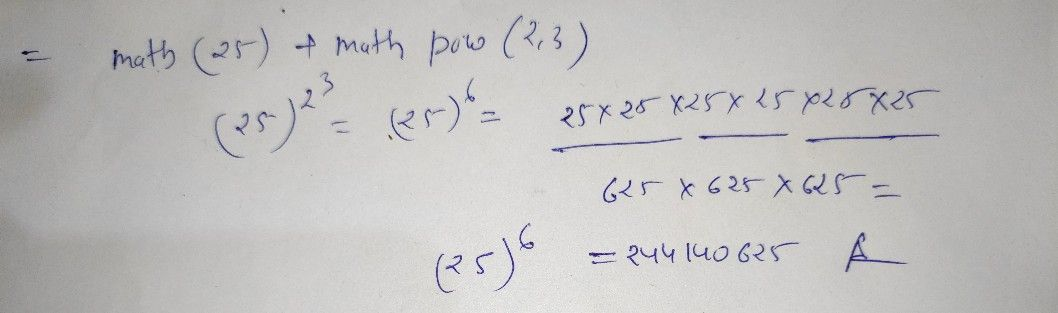Symbol
Problem$\left(25\right)+M$ $2$ $2.Matn.sg\pi \left(25\right)+Matn.pOM\left(2,3\right)$ $M\left(2,3\right)$